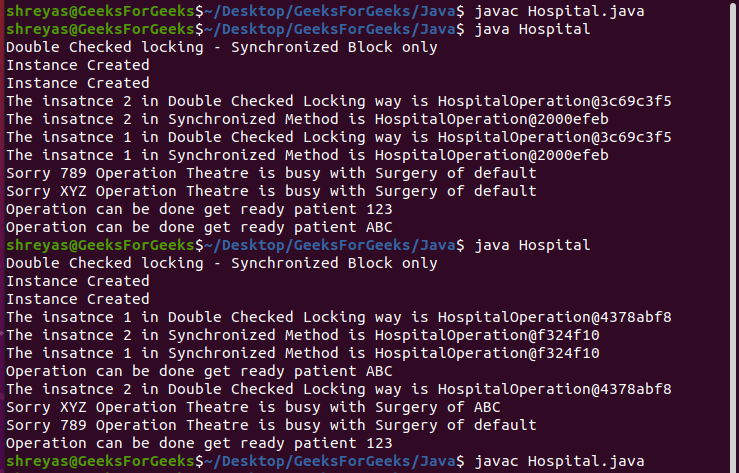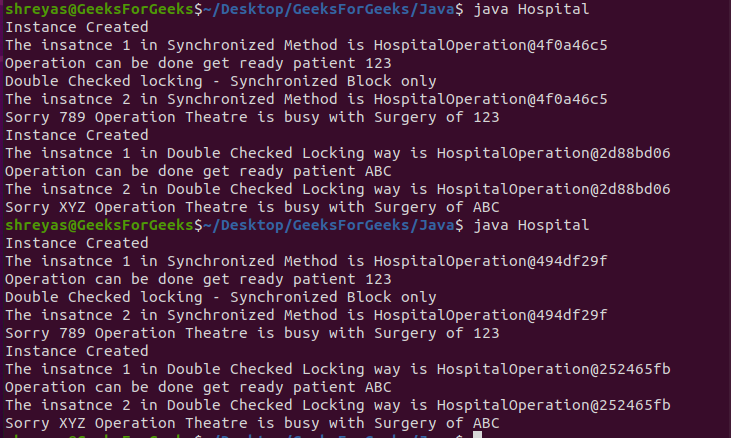# Java Program to Demonstrate the Lazy Initialization Thread-Safe

• Last Updated : 14 Feb, 2022

Java is a popular object-oriented programming language used by developers and coders for website/app development. The creation of a class object using a new keyword is referred to as object instantiation. Java by default allows users to define two ways of object instantiation which are Eager and Lazy. Java Interpreter interprets program/script code line by line while it is executed, but java Compiler compiles the complete program before starting the Interpretation. In eager instantiation, the object creation occurs at compile-time, so it is not considered efficient enough when we have large programs or many object instantiations as those might not be used. Lazy Instantiation means instantiating an object only when it is needed. This is considered efficient as it saves space and processing power when we consider large programs or many object instantiations.

Threads are considered as small individual components of processing that can execute in a parallel fashion to enhance/fasten the computation time. With advanced multi-core computers, threading has found a wide range of applications in programming. In the context of java, a thread-safe code is one that doesn’t provide anomalous output irrespective of how many times it is executed. Thread-safe code is very much essential for avoiding programming defects/flaws like race conditions, deadlocks, etc.

Methods:

Back to lazy Instantiation which is often interchangeably referred to as on-demand instantiation has 2 methods of implementing itself they are  as follows:

1. Using synchronized keywords on the entire method/function is good when the method size is small and not many threads are going to access it soon as it is computation processing heavy.
2. The next and more efficient way is the synchronized block way in which you just wrap around that code which needs to be thread-safe and to ensure more surety we wrap it with double-checked locking. The following approach of the hospital case study will help better understand these concepts in practical applications

Approach:

The following is the approach followed in the script to demonstrate Lazy instantiation in a thread-safe environment (both synchronized method and synchronized block i.e. double-checked locking is covered) with the help of hospital-Operation case study is as follows:

1. Assume there is a hospital that has potentially 1 operation theater wherein the surgeries would be performed on patients.
2. The catch here is we would like to create a Hospital class object only when it is needed (when there is a patient whose operation needs to be performed) else the Hospital object would not be created — this is referred to as lazy Instantiation
3. In order to demonstrate a multi-threaded environment, we are having various threads (t1, t2, t3, and t4) which are illustrated as patients.
4. So let say if a patient whose operation needs to be performed we make Hospital object then and call operation() method which takes in the patient name as an argument.
5. Then if the operation theater is empty or not the appropriate message would be displayed as to get ready for operation or whose surgery is currently being performed respectively.
6. To make things more realistic and explain the concept of double-checked locking we are demonstrating the two ways of Lazy Instantiations those are synchronized methods (getInstanceSynchronizedWay()) which as explained above is a little less efficient/more expensive than the synchronized block way that is getInstanceSynchronizedBlockWay()
7. Also, there are a few Thread.sleep() commenting inside the code for the purpose of demonstrating the thread-safety/serial execution of threads and tracking output is a controlled manner which would be further explained in the explanation Section.

Implementation:

The following is the scenario in which there is a hospital with only one operation theatre. So if the operation theatre is empty then take the patient for operation else display the sorry operation is carried for another patient. There are multiple threads created in this script with the illustration of each thread as a patient.

Example:

## Java

 `// Java Program to Demonstration of Lazy Instantiation in``// Thread-Safe Environment Using synchronized method and``// Double-Checked Locking` `// Class 1``// Helper class acting as Singleton Class``class` `HospitalOperation {` `    ``// Private class variables``    ``private` `static` `HospitalOperation _instance;``    ``private` `static` `HospitalOperation``        ``_instanceForDoubleCheckLocking;``    ``private` `boolean` `empty = ``false``;``    ``private` `String patientName = ``"default"``;` `    ``// Method 1``    ``// Displays Instance created only when new Instance is``    ``// Created``    ``private` `HospitalOperation()``    ``{``        ``System.out.println(``"Instance Created"``);``    ``}` `    ``// Method 2``    ``// Synchronized method() Approach``    ``public` `static` `synchronized` `HospitalOperation``    ``getInstanceSynchronizedWay()``    ``{` `        ``if` `(_instance == ``null``)``            ``_instance = ``new` `HospitalOperation();` `        ``return` `_instance;``    ``}` `    ``// Method 3``    ``// Double Checked Locking- Synchronized Block``    ``public` `static` `HospitalOperation``    ``getInstanceSynchronizedBlockWay()``    ``{` `        ``// Checking for double locking``        ``if` `(_instanceForDoubleCheckLocking == ``null``)``            ``synchronized` `(HospitalOperation.``class``)``            ``{``                ``if` `(_instanceForDoubleCheckLocking == ``null``)``                    ``_instanceForDoubleCheckLocking``                        ``= ``new` `HospitalOperation();``            ``}` `        ``return` `_instanceForDoubleCheckLocking;``    ``}` `    ``// Method 4``    ``// Checks if operation theatre is empty or not``    ``public` `boolean` `isOperationTheatreEmpty()``    ``{``        ``return` `empty;``    ``}` `    ``// Method 5``    ``// Called when Operation is finished``    ``public` `void` `endOperation() { empty = ``true``; }` `    ``// Method 6``    ``// Accessed by more than one threads``    ``public` `synchronized` `void` `operation(String aName)``    ``{` `        ``// When flag variables changes from false to true``        ``if` `(empty == ``true``) {``            ``patientName = aName;` `            ``// Get the patient ready as operation can be``            ``// performed``            ``System.out.println(``"Operation can be done "``                               ``+ ``"get ready patient "``                               ``+ patientName);``            ``empty = ``false``;``        ``}` `        ``// Operation can not be performed``        ``else` `{``            ``// Print and display``            ``System.out.println(``                ``"Sorry "` `+ aName``                ``+ ``" Operation Theatre is busy with Surgery of "``                ``+ patientName);``        ``}``    ``}``}` `// Class 2``// Main class``public` `class` `Hospital {` `    ``// Main driver method``    ``public` `static` `void` `main(String args[])``    ``{` `        ``// Synchronized method` `        ``// Now creating a thread in main() method``        ``Thread t1 = ``new` `Thread(``new` `Runnable() {``            ``// run() method for this thread``            ``public` `void` `run()``            ``{` `                ``// Creating object of above class in``                ``// this class main() method``                ``HospitalOperation i1``                    ``= HospitalOperation``                          ``.getInstanceSynchronizedWay();` `                ``// Print statement only``                ``System.out.println(``                    ``"The instance 1 in Synchronized Method is "``                    ``+ i1);` `                ``// Calling the method``                ``// passing custom argument as input``                ``i1.endOperation();``                ``i1.operation(``"123"``);``            ``}``        ``});` `        ``// Thread 2``        ``// Again creating another thread``        ``Thread t2 = ``new` `Thread(``new` `Runnable() {``            ``// run() method for this thread``            ``public` `void` `run()``            ``{` `                ``HospitalOperation i2``                    ``= HospitalOperation``                          ``.getInstanceSynchronizedWay();` `                ``System.out.println(``                    ``"The instance 2 in Synchronized Method is "``                    ``+ i2);``                ``i2.operation(``"789"``);``            ``}``        ``});` `        ``// We delay thread also to ensure that``        ``// sequence of output is correct` `        ``// Starting the first thread``        ``// using start() method for threads``        ``t1.start();` `        ``// try {``        ``//     Thread.sleep(1000);``        ``// }``        ``// catch (InterruptedException e)``        ``//     {}` `        ``//  Similarly, starting the second thread``        ``t2.start();` `        ``// Double Checked Locking` `        ``// Print statement only``        ``System.out.println(``            ``"Double Checked locking - Synchronized Block only"``);` `        ``// Thread 3``        ``// Again creating a thread using runnable interface``        ``Thread t3 = ``new` `Thread(``new` `Runnable() {``            ``// run() method for this thread``            ``public` `void` `run()``            ``{` `                ``HospitalOperation i1``                    ``= HospitalOperation``                          ``.getInstanceSynchronizedBlockWay();` `                ``System.out.println(``                    ``"The instance 1 in Double Checked Locking way is "``                    ``+ i1);` `                ``i1.endOperation();``                ``i1.operation(``"ABC"``);``            ``}``        ``});` `        ``// Thread 4``        ``// LAstly creating another thread``        ``Thread t4 = ``new` `Thread(``new` `Runnable() {``            ``// run() method for this thread``            ``public` `void` `run()``            ``{``                ``HospitalOperation i2``                    ``= HospitalOperation``                          ``.getInstanceSynchronizedBlockWay();` `                ``System.out.println(``                    ``"The instance 2 in Double Checked Locking way is "``                    ``+ i2);` `                ``i2.operation(``"XYZ"``);``            ``}``        ``});``        ``// We delay thread also to ensure that``        ``// sequence of output is correct``        ``// try {``        ``//     Thread.sleep(1000);``        ``// }``        ``// catch (InterruptedException e)``        ``//     {}``        ``t3.start();``        ``// try {``        ``//     Thread.sleep(1000);``        ``// }``        ``// catch (InterruptedException e)``        ``//     {}``        ``t4.start();``    ``}``}`

Explanation: The following is the explanation of the above case study Hospital with one operation theater,

1. Initially, 2 threads namely t1 and t2 (illustrated as patients) are created in the main() method (inside Hospital class) and then those threads are running simultaneously with the program.
2. Then these threads call the Synchronized Method (getInstanceSynchronizedBlockWay()) way to create/use the Lazy Instantiation of HospitalOperation class to perform an operation on patients named 123 and 789 respectively.
3. Initially say default patient’s operation/surgery was going through in the operation theater. So until endOperation() method is not called prior to operation() method, it going to be busy and display Sorry message accordingly to the patient named 789 (thread t2) but for patient name 123 (thread t1) as endOperation() method is called prior to the operation()  method call, 123 would be accepted for the surgery in the operation displaying the appropriate message as soon in the output screenshots.
4. Exactly same process as above is repeated for patients ABC and XYZ (threads t1 and t2) to demonstrate the concept of double-checked locking that is a synchronized block way of doing Lazy Instantiation of the object.
5. One thing to notice is the object id of HospitalOperation class is printed for t1 and t2 which is the same as they are demonstrating the synchronized method approach and object id is same for t3 and t4 is same as well as they are demonstrating synchronized block method which makes clear that HospitalOperation class acts as a Singleton class for each strategy of lazy instantiation types.
6. Also, when the Thread.sleep() methods are un-commented from the code then the output occurs almost like threads are processing serially and not in a parallel fashion which ensures the same business logic (comparing figure 1 and 2 of sample output screenshots) is found in the output which demonstrates that the script is thread-safe.

Output: